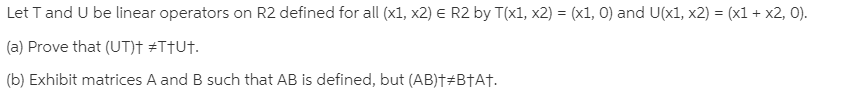# Let T and U be linear operators on R2 defined for all (x1, x2) E R2 by T(x1, x2) = (x1, 0) and U(x1, x2) = (x1 + x2, 0).(a) Prove that (UT)† #TTU†.(b) Exhibit matrices A and B such that AB is defined, but (AB)†#B†A†.

Question
1 viewshelp_outlineImage TranscriptioncloseLet T and U be linear operators on R2 defined for all (x1, x2) E R2 by T(x1, x2) = (x1, 0) and U(x1, x2) = (x1 + x2, 0). (a) Prove that (UT)† #TTU†. (b) Exhibit matrices A and B such that AB is defined, but (AB)†#B†A†. fullscreen

### This question hasn't been answered yet.